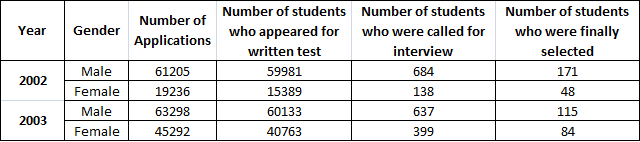### CAT 2003(L) Question 3

Instructions

DIRECTIONS for the following three questions: In each question, there are two statements: A and B, either of which can be true or false on the basis of the information given below.

A research agency collected the following data regarding the admission process of a reputed management school in India.Question 3

# Statement A: The percentage of absentees in the written test among females decreased from 2002 to 2003.Statement B: The percentage of absentees in the written test among males was larger than among females in 2003.

Solution

The percentage of absentees in the written test among females in 2002 was 3847/19236 and in 2003 was 4529/45292. Thus  percentage of absentees decreased from 02 to 03. Hence statement A is true.

The percentage of absentees in the written test among males in 2003 is 3065/63298 which is clearly less than that of female in 2003. Hence statement b is false . Hence option A.